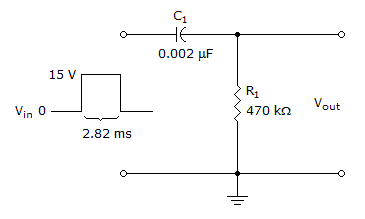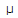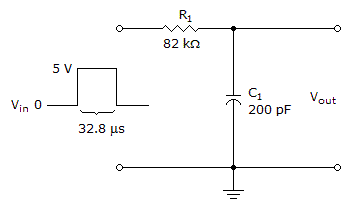# Electronics - Time Response of Reactive Circuits

### Exercise :: Time Response of Reactive Circuits - General Questions

6.In the given circuit, what must the pulse width and time between pulses be to allow the capacitor to completely charge by the end of each pulse and to completely discharge between each pulse?

 A. 940s B. 2.82 ms C. 3.76 ms D. 4.7 ms

Answer: Option D

Explanation:

No answer description available for this question. Let us discuss.

7.The given circuit is an

 A. RL integrator B. RC differentiator C. RL differentiator D. RC integrator

Answer: Option D

Explanation:

No answer description available for this question. Let us discuss.

8.

Which of the following is true for a capacitor?

 A. A capacitor acts like a short to instantaneous changes in current. B. A capacitor's voltage cannot change instantaneously. C. A capacitor acts like an open to dc. D. All of the above

Answer: Option D

Explanation:

No answer description available for this question. Let us discuss.

9.

If the capacitor in an RC integrator shorts, the output

 A. is at ground B. would measure the same as the input C. would measure zero volts D. None of the above

Answer: Option A

Explanation:

No answer description available for this question. Let us discuss.

10.What has the voltage across the resistor decayed to by the end of the pulse in the given circuit?

 A. 0 V B. 0.75 V C. 5.55 V D. 14.25 V

Answer: Option B

Explanation:

No answer description available for this question. Let us discuss.

#### Current Affairs 2021

Interview Questions and Answers